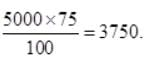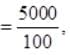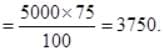Test: Operations on Large Numbers- 2

# Test: Operations on Large Numbers- 2

Test Description

## 10 Questions MCQ Test Mathematics for Class 5 | Test: Operations on Large Numbers- 2

Test: Operations on Large Numbers- 2 for Class 5 2022 is part of Mathematics for Class 5 preparation. The Test: Operations on Large Numbers- 2 questions and answers have been prepared according to the Class 5 exam syllabus.The Test: Operations on Large Numbers- 2 MCQs are made for Class 5 2022 Exam. Find important definitions, questions, notes, meanings, examples, exercises, MCQs and online tests for Test: Operations on Large Numbers- 2 below.
Solutions of Test: Operations on Large Numbers- 2 questions in English are available as part of our Mathematics for Class 5 for Class 5 & Test: Operations on Large Numbers- 2 solutions in Hindi for Mathematics for Class 5 course. Download more important topics, notes, lectures and mock test series for Class 5 Exam by signing up for free. Attempt Test: Operations on Large Numbers- 2 | 10 questions in 15 minutes | Mock test for Class 5 preparation | Free important questions MCQ to study Mathematics for Class 5 for Class 5 Exam | Download free PDF with solutions
 1 Crore+ students have signed up on EduRev. Have you?
Test: Operations on Large Numbers- 2 - Question 1

### Read the statements and choose the correct option: Statement A:  3 x 15 = 15 x 3 Statement B: 3 ÷ 2 = 2 ÷ 3

Detailed Solution for Test: Operations on Large Numbers- 2 - Question 1

3 x 15 = 45 and also 15 x 3 = 45. Thus A is true. 3 ÷ 2 = 1.5 and 2 ÷ 3 = 0.66 which are not equal. Therefore only statement A is true.

Test: Operations on Large Numbers- 2 - Question 2

### If there are 5000 mangoes in 100 boxes, how many mangoes will be there in 75 boxes?

Detailed Solution for Test: Operations on Large Numbers- 2 - Question 2

Mangoes in 100 boxes =Mangoes in 1 boxMangoes in 75 boxesTest: Operations on Large Numbers- 2 - Question 3

### If Fatima has an equal number of notes of denomination 10, 5 and 2 and the total amount she has Rs. 510, then the number of notes of each she had

Detailed Solution for Test: Operations on Large Numbers- 2 - Question 3

Let the number of notes of each kind be x. Then 10x + 5x + 2x = 510 ⇒ x = 510 / 17 = 30.

Test: Operations on Large Numbers- 2 - Question 4

There are 1,82,039 roses in a garden. If one rose out of every 13 roses is spoiled, then the total number of spoiled roses, is

Detailed Solution for Test: Operations on Large Numbers- 2 - Question 4

No. of spoiled roses = 182039 ÷ 13 = 14,003

Test: Operations on Large Numbers- 2 - Question 5

Directions: Use the MULTIPLICATION OPERATION and evaluate the following questions.
24 x 65

Detailed Solution for Test: Operations on Large Numbers- 2 - Question 5

24 x 65 = 1560

Test: Operations on Large Numbers- 2 - Question 6

Directions: Use the MULTIPLICATION OPERATION and evaluate the following questions.
1042 x 5

Detailed Solution for Test: Operations on Large Numbers- 2 - Question 6

​1042 x 5 = 5210

Test: Operations on Large Numbers- 2 - Question 7

Directions: Use the ADDITION OPERATION and evaluate the following questions.
881 + 88

Detailed Solution for Test: Operations on Large Numbers- 2 - Question 7

881 + 88 = 969

Test: Operations on Large Numbers- 2 - Question 8

Directions: Use the ADDITION OPERATION and evaluate the following questions.
2547 + 9998

Detailed Solution for Test: Operations on Large Numbers- 2 - Question 8

2547 + 9998 = 12545

Test: Operations on Large Numbers- 2 - Question 9

Directions: Use the ADDITION OPERATION and evaluate the following questions.
Look at these numbers: 0.2 2.5 2.66.1
Choose two numbers from the box to complete the addition sentence. ____+_____=2.7

Detailed Solution for Test: Operations on Large Numbers- 2 - Question 9

Look for numbers in the box that are less than 2.7.

• Try 0.2. Now we have to find the number which when added to 0.2 gives 2.7.
• That number is 2.5. thus 2.5 + 0.2 = 2.7.
Test: Operations on Large Numbers- 2 - Question 10

Directions: Use the ADDITION OPERATION and evaluate the following questions.
428.65 + 500.5

Detailed Solution for Test: Operations on Large Numbers- 2 - Question 10

428.65 + 500.5 = 929.15 [Since 500.5 = 500.50]

## Mathematics for Class 5

9 videos|54 docs|35 tests
 Use Code STAYHOME200 and get INR 200 additional OFF Use Coupon Code
Information about Test: Operations on Large Numbers- 2 Page
In this test you can find the Exam questions for Test: Operations on Large Numbers- 2 solved & explained in the simplest way possible. Besides giving Questions and answers for Test: Operations on Large Numbers- 2, EduRev gives you an ample number of Online tests for practice

## Mathematics for Class 5

9 videos|54 docs|35 tests